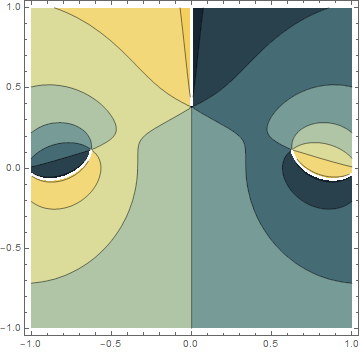# Paul Klee meets Perry the Platypus

I was playing around with something in Mathematica and one of the images that came out of it surprised me.It’s a contour plot for the system function of a low pass filter.

```    H[z_] := 0.05634*(1 + 1/z)*(1 - 1.0166/z + 1/z^2) /
((1 - 0.683/z)*(1 - 1.4461/z + 0.7957/z^2))
ContourPlot[ Arg[H[Exp[I (x + I y)]]],
{x, -1, 1}, {y, -1, 1},
ColorFunction -> "StarryNightColors"]
```

It looks sorta like a cross between Paul Klee’s painting Senecioand Perry the Platypus from Phineas and Ferb.## One thought on “Paul Klee meets Perry the Platypus”

1. How surprized I was after plotting y^8 -12*y^7 +6*x^2*y^6 +60*y^6 -72*x^2*y^5 -180*y^5 +169*x^4*y^4 +306*x^2*y^4 +373*y^4 -108*x^4*y^3 -432*x^2*y^3 -648*y^3 +960*x^6*y^2 +1446*x^4*y^2 -678*x^2*y^2 +294*y^2 -972*x^4*y +1944*x^2*y +1440*x^8 -2880*x^6 +441*x^4 -882*x^2 -18